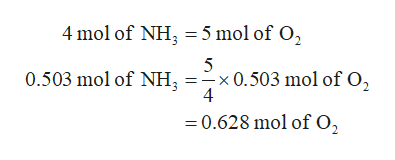# For the following reaction, if 0.503 mole NH3 reacts with 0.356 mole O2, which is the limiting reagent for the product NO? (Balance Equation)NH3 (g) + O2 (g)---- NO (g) + H2O (l)

Question
2 views

For the following reaction, if 0.503 mole NH3 reacts with 0.356 mole O2, which is the limiting reagent for the product NO? (Balance Equation)

NH3 (g) + O2 (g)---- NO (g) + H2O (l)

check_circle

Step 1

The given reaction is shown below.

Step 2

The given reaction is unbalanced; the number of hydrogen atom in reactant side is 3, whereas in product side is 2. Therefore, the first step is to balance the given reaction by multiplying it with some coefficient as shown below.

Step 3

It is given that 0.503 mole of NH3 reacts with 0.356 mole of O2 to form the desired product. From the reaction, it is clear that 4 mole of ammonia reacts with...help_outlineImage Transcriptionclose4 mol of NH =5 mol of O2 x0.503 mol of O2 4 0.503 mol of NH =0.628 mol of O, fullscreen

### Want to see the full answer?

See Solution

#### Want to see this answer and more?

Solutions are written by subject experts who are available 24/7. Questions are typically answered within 1 hour.*

See Solution
*Response times may vary by subject and question.
Tagged in

### Other